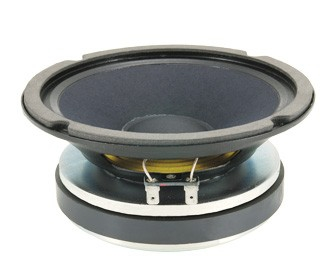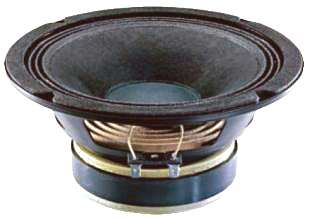## Ciare

Ciare is a well known Italian manufacturer of high quality PA drivers as well as car and hi-fi drivers.PM 120       order no. C-PM120- not available
11 cm full range driver with paper cone.
• power handling (continuous/programme) = 30/60 W
• resonance frequency fs = 117 Hz
• impedance R = 8 Ohm
• sound pressure level SPL = 85 dB (2,83V; 1m)
• DC resistance Re = 7,6 Ohm
• force factor BL = 2,8 N/A
• voice coil inductance L = 0,07 mH
• effective mechanical mass incl. air load mms = 4,2 g
• equivalent volume of compliance Vas = 4 l
• total Q factor Qts = 1,82 (Qms=4,69, Qes=2,98)
• voice coil diameter = 20 mm
• maximum peak linear excursion vibration xlin = +/- 0,75 mm
• mounting diameter d = 109 mm
• overall diameter d = 120 mm
• mounting depth (not countersunk) t = 49 mm
• mass m = 0,34 kg
PDF data sheetrecommended cabinet 1:
closed cabinet with 2,9 L volume
from 638/403 Hz (-3dB/-8dB)

recommended cabinet 2:
10,6 L volume bass reflex cabinet
with 4x HP100 reflex tube, -6 cm long.
from 323/257 Hz (-3dB/-8dB).

6.38MR3 (639401)       order no. C-35040       EUR 66,00excl. VAT: € 55.46 / \$ 62.67
15 cm midrange driver with paper cone. The speaker has soldering lugs / faston terminals.
• power handling (continuous/programme) = 100/200 W
• frequency range = 500-5000 Hz
• resonance frequency fs = 302,05 Hz
• impedance R = 8 Ohm
• sound pressure level SPL = 102 dB (2,83V; 1m)
• DC resistance Re = 5,5 Ohm
• force factor BL = 10,93 N/A
• voice coil inductance L = 0,23 mH
• effective piston radiating area Sd = 141,03 cm2
• effective mechanical mass incl. air load mms = 6,37 g
• equivalent volume of compliance Vas = 12,2 l
• total Q factor Qts = 0,38 (Qms=1,35, Qes=0,53)
• voice coil diameter = 38 mm
• maximum peak linear excursion vibration xlin = +/- 0,25 mm
• mounting diameter d = 143 mm
• overall diameter d = 165 mm
• mounting depth (not countersunk) t = 76 mm
• mass m = 2,8 kg
PDF data sheetrecommended cabinet 1:
closed cabinet with 2,8 L volume
from 194/122 Hz (-3dB/-8dB)

recommended cabinet 2:
9,1 L volume bass reflex cabinet
with HP70 reflex tube, 7 cm long.
from 103/80 Hz (-3dB/-8dB).

PM 200       order no. C-PM200       EUR 103,64excl. VAT: € 87.09 / \$ 98.41
19 cm full range driver with paper cone.
• power handling (continuous/programme) = 120/250 W
• resonance frequency fs = 80 Hz
• impedance R = 8 Ohm
• sound pressure level SPL = 96 dB (2,83V; 1m)
• DC resistance Re = 6,6 Ohm
• force factor BL = 11,2 N/A
• voice coil inductance L = 0,43 mH
• effective mechanical mass incl. air load mms = 15 g
• equivalent volume of compliance Vas = 16,6 l
• total Q factor Qts = 0,33 (Qms=1,68, Qes=0,41)
• voice coil diameter = 38 mm
• maximum peak linear excursion vibration xlin = +/- 1,5 mm
• mounting diameter d = 180 mm
• overall diameter d = 209,5 mm
• mounting depth (not countersunk) t = 90,5 mm
• mass m = 3 kg
PDF data sheetrecommended cabinet 1:
closed cabinet with 6,7 L volume
from 136/86 Hz (-3dB/-8dB)

recommended cabinet 2:
27 L volume bass reflex cabinet
with HP100 reflex tube, 10 cm long.
from 62/53 Hz (-3dB/-8dB).

PM 203       order no. C-PM203       EUR 122,10excl. VAT: € 102.61 / \$ 115.94
20 cm full range driver with paper cone.
• power handling (continuous/programme) = 150/300 W
• resonance frequency fs = 91 Hz
• impedance R = 8 Ohm
• sound pressure level SPL = 94 dB (2,83V; 1m)
• DC resistance Re = 5,6 Ohm
• force factor BL = 10,09 N/A
• voice coil inductance L = 0,58 mH
• effective mechanical mass incl. air load mms = 20,2 g
• equivalent volume of compliance Vas = 10 l
• total Q factor Qts = 0,54 (Qms=3,28, Qes=0,64)
• voice coil diameter = 50 mm
• maximum peak linear excursion vibration xlin = +/- 2,5 mm
• mounting diameter d = 180 mm
• overall diameter d = 210 mm
• mounting depth (not countersunk) t = 96 mm
• mass m = 2,6 kg
PDF data sheet

## Wooferrecommended cabinet 1:
closed cabinet with 14,5 L volume
from 120/76 Hz (-3dB/-8dB)

recommended cabinet 2:
45 L volume bass reflex cabinet
with 2x HP100 reflex tube, 16 cm long.
from 65/50 Hz (-3dB/-8dB).

PW 328       order no. C-PW328       EUR 151,92excl. VAT: € 127.66 / \$ 144.26
30 cm midrange driver with paper cone.
• power handling (continuous/programme) = 150/300 W
• resonance frequency fs = 48 Hz
• impedance R = 8 Ohm
• sound pressure level SPL = 98 dB (2,83V; 1m)
• DC resistance Re = 5,52 Ohm
• force factor BL = 15,01 N/A
• voice coil inductance L = 0,82 mH
• effective mechanical mass incl. air load mms = 48,5 g
• equivalent volume of compliance Vas = 91 l
• total Q factor Qts = 0,32 (Qms=3,08, Qes=0,36)
• voice coil diameter = 50 mm
• maximum peak linear excursion vibration xlin = +/- 3 mm
• mounting diameter d = 282 mm
• overall diameter d = 315 mm
• mounting depth (not countersunk) t = 128 mm
• mass m = 3,7 kg
PDF data sheetrecommended cabinet 1:
closed cabinet with 7,7 L volume
from 156/99 Hz (-3dB/-8dB)

recommended cabinet 2:
21 L volume bass reflex cabinet
with 2x HP100 reflex tube, 23 cm long.
from 89/68 Hz (-3dB/-8dB).

PW 330       order no. C-PW330       EUR 425,93excl. VAT: € 357.92 / \$ 404.45
30 cm midrange driver with paper cone.
• power handling (continuous/programme) = 500/1000 W
• resonance frequency fs = 55 Hz
• impedance R = 8 Ohm
• sound pressure level SPL = 100 dB (2,83V; 1m)
• DC resistance Re = 5,33 Ohm
• force factor BL = 18,69 N/A
• voice coil inductance L = 0,45 mH
• effective mechanical mass incl. air load mms = 54,9 g
• equivalent volume of compliance Vas = 65 l
• total Q factor Qts = 0,28 (Qms=10,33, Qes=0,29)
• voice coil diameter = 100 mm
• maximum peak linear excursion vibration xlin = +/- 3,75 mm
• mounting diameter d = 282 mm
• overall diameter d = 315 mm
• mounting depth (not countersunk) t = 145 mm
• mass m = 11,1 kg
PDF data sheetrecommended cabinet 1:
closed cabinet with 14,5 L volume
from 120/76 Hz (-3dB/-8dB)

recommended cabinet 2:
45 L volume bass reflex cabinet
with 2x HP100 reflex tube, 16 cm long.
from 65/50 Hz (-3dB/-8dB).

PW 328       order no. C-PW328       EUR 151,92excl. VAT: € 127.66 / \$ 144.26
30 cm midrange driver with paper cone.
• power handling (continuous/programme) = 150/300 W
• resonance frequency fs = 48 Hz
• impedance R = 8 Ohm
• sound pressure level SPL = 98 dB (2,83V; 1m)
• DC resistance Re = 5,52 Ohm
• force factor BL = 15,01 N/A
• voice coil inductance L = 0,82 mH
• effective mechanical mass incl. air load mms = 48,5 g
• equivalent volume of compliance Vas = 91 l
• total Q factor Qts = 0,32 (Qms=3,08, Qes=0,36)
• voice coil diameter = 50 mm
• maximum peak linear excursion vibration xlin = +/- 3 mm
• mounting diameter d = 282 mm
• overall diameter d = 315 mm
• mounting depth (not countersunk) t = 128 mm
• mass m = 3,7 kg
PDF data sheetrecommended cabinet 1:
closed cabinet with 7,7 L volume
from 156/99 Hz (-3dB/-8dB)

recommended cabinet 2:
21 L volume bass reflex cabinet
with 2x HP100 reflex tube, 23 cm long.
from 89/68 Hz (-3dB/-8dB).

PW 330       order no. C-PW330       EUR 425,93excl. VAT: € 357.92 / \$ 404.45
30 cm midrange driver with paper cone.
• power handling (continuous/programme) = 500/1000 W
• resonance frequency fs = 55 Hz
• impedance R = 8 Ohm
• sound pressure level SPL = 100 dB (2,83V; 1m)
• DC resistance Re = 5,33 Ohm
• force factor BL = 18,69 N/A
• voice coil inductance L = 0,45 mH
• effective mechanical mass incl. air load mms = 54,9 g
• equivalent volume of compliance Vas = 65 l
• total Q factor Qts = 0,28 (Qms=10,33, Qes=0,29)
• voice coil diameter = 100 mm
• maximum peak linear excursion vibration xlin = +/- 3,75 mm
• mounting diameter d = 282 mm
• overall diameter d = 315 mm
• mounting depth (not countersunk) t = 145 mm
• mass m = 11,1 kg
PDF data sheet

The cabinet volume calculations are executed considering an external resistance of 0.3 Ohm. We are using, if no other data is known or available, the information supplied by the manufacturer.

Please note: the frequency response doesn't say much about the cut-off frequency inside the cabinet! Therefore, we mostly give you 2 examples with dimensioning considerations and an indication, how low the loudspeaker will reproduce.

Home